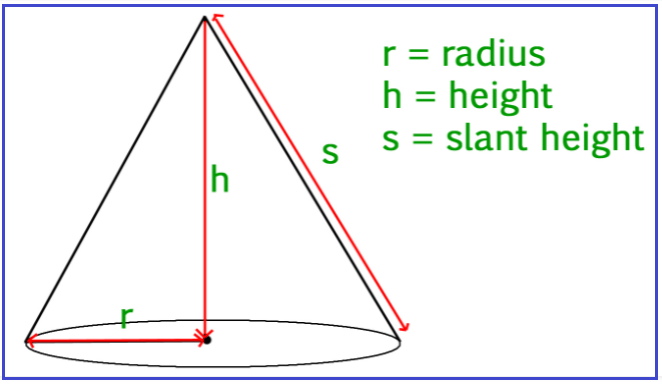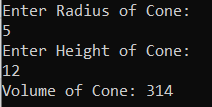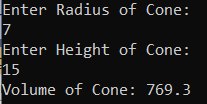# Volume of Cone in C#

## The Volume of Cone in C# with Examples

In this article, I am going to discuss how to calculate the Volume of Cone in C# with Examples. Please read our previous article, where we discussed How to Calculate the Surface Area of the Cone in C#. Here, in this article, first, we will discuss what is a Cone, then we will discuss how to calculate the volume of the Cone and finally, we will see how to implement the Volume of Cone program in C#.

##### What is a Cone?

According to Wikipedia, A cone is a three-dimensional geometric shape that tapers smoothly from a flat base to a point called the apex or vertex. A cone is formed by a set of line segments, half-lines, or lines connecting a common point, the apex, to all of the points on a base that is in a plane that does not contain the apex.##### The volume of Cone:

The volume of a cone defines the space or the capacity of the cone.
The volume of a cone = (1/3) πr2h cubic units
Where r is the base radius of the cone, s is the slant height of a cone and h is the height of the cone

###### Example:

r = 2, h= 5
The volume of a cone = (1/3) πr2h cubic units
V= (1/3) × 3.14 × 22 ×5
V= (1/3) × 3.14 × 4 ×5
V= (1/3) × 3.14 × 20
V = 20.93 cm3

##### Logic to Calculate the Volume of Cone in C#

To find the Volume of the Cone, we need to follow the below steps

1. We will define Radius, Height, and Volume variables.
2. Then we will assign the value of Radius, and Height.
3. Then we will calculate the volume of the cone by using this formula (1/3) πr2h, where, π =3.14
4. And the resulting output will store in the Volume variable.
##### Example: Volume of Cone Program in C#.

The following C# Program will calculate the Volume of the Cone.

```using System;
public class VolumeOfCone
{
public static void Main()
{
float Height = 5;
double PI = 3.14;
double Volume = (1.0/3) * PI * Height * Radius * Radius;
Console.WriteLine("Volume of Cone: " + Volume);
}
}
```

Output: Volume of Cone: 20.9333333333333

##### Example: Taking input from the user

In the following C# program, we will take the cone radius and height from the user and then calculate the Volume and print it on the console.

```using System;
public class VolumeOfCone
{
public static void Main()
{
Console.WriteLine("Enter Height of Cone:");

double PI = 3.14;
double Volume = (1.0/3) * PI * Height * Radius * Radius;
Console.WriteLine("Volume of Cone: " + Volume);
}
}
```
###### Output:##### C# Code (Using function)

In the below example, we have created one method to calculate the volume of a cone.

```using System;
public class VolumeOfCone
{
public static void Main()
{
Console.WriteLine("Enter Height of Cone:");
Console.WriteLine("Volume of Cone: " + Volume);
}

private static double CalculateVolume(double Radius, double Height)
{
double PI = 3.14;
double Volume = (1.0 / 3) * PI * Height * Radius * Radius;
return Volume;
}
}
```
###### Output:In the next article, I am going to discuss Celsius to Fahrenheit in C# with Examples. Here, in this article, I try to explain how to calculate the Volume of Cone in C# with Examples and I hope you enjoy this Volume of Cone Program article.